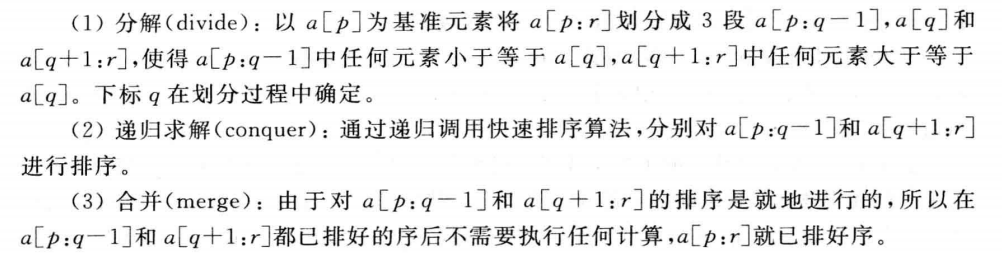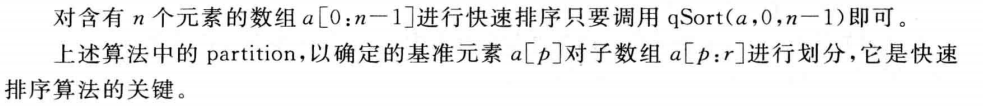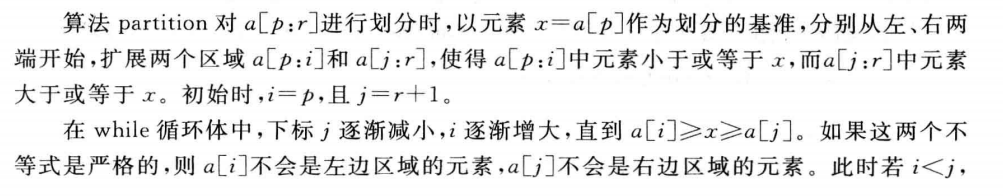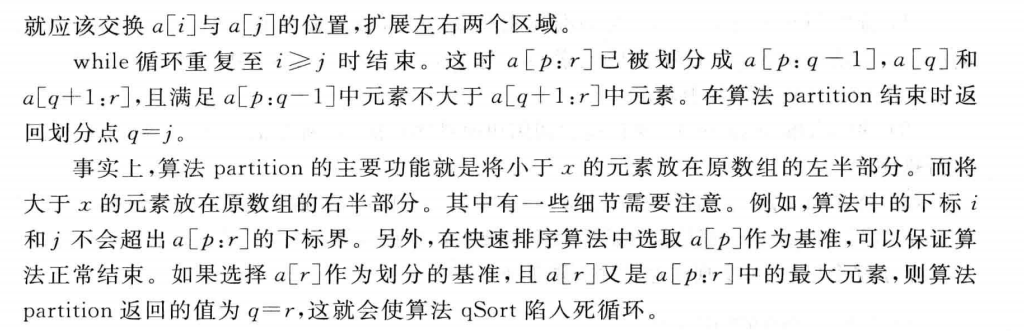## 算法设计与分析——快速排序```private static void qSort(int p,int r)
{
if(p<r)
{
int q = partition(p,r);
qSort(p,q-1);//对左半段排序
qSort(q+1,r);//对右半段排序
}
}``````private static int partition(int p,int r)
{
int i = p;
int j = r + 1;
Comparable x = a[p];
//将<x的元素交换到左边区域
//将>x的元素交换到右边区域
while(true)
{
while(a[++i].compareTo(x)<0&&i<r);
while(a[--j].compareTo(x)>0)；
if(i>=j) break;
MyMath.swap(a,i,j);
}
a[p]=a[j];
a[j]=x;
return j;
}```在这里解释一下为什么会出现死循环？如果选择a[p]作为划分的基准是，a[p]是a[p:r]中的最小元素为什么不会陷入死循环？